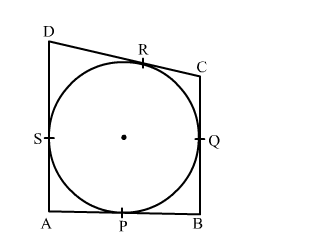# In the given figure, a quadrilateral ABCD is drawn to circumscribe a circle such that its side AB,`
Question:

In the given figure, a quadrilateral ABCD is drawn to circumscribe a circle such that its side AB, BC, CD and AD touch the circle at P, Q, R and S respectively. If AB = x cm, BC = 7 cm, CR = 3 cm and AS = 5 cm , find xSolution:

We know that tangent segments to a circle from the same external point are congruent.
Now, we have
CR = CQ, AS = AP and BQ = BP
Now, BC = 7 cm
⇒ CQ + BQ = 7
⇒ BQ = 7 − CQ
⇒ BQ = 7 − 3          [∵ CQ = CR = 3]
⇒ BQ = 4 cm
Again, AB = AP + PB
= AP + BQ
= 5 + 4                           [∵ AS = AP = 5]
= 9 cm
Hence, the value of x is 9 cm.